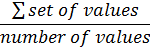### Averages: Mean, Median and Mode

Averages give us the "common" value in a set of data. There are 3 ways to measure the average:

1.  Mean
is the most commonly used average. It is calculated by adding up the set of values and then dividing that sum, by the number of values used:
 Mean =Where the symbol Σ is sum

2.  Median
order the set of values by size then work out the middle
value

3.  Mode
the value that occurs most often

#### Example 1.   Find the mean, median and mode of15   19   10   17   17   16   18

Median
(a) order the numbers
10, 15, 16, 17, 17, 18, 19
(b) find the median which is the middle number:
10, 15, 16, 17, 17, 18, 19 = 17

Mode find the most common value: 17 occurs twice so it is 17

#### Example 2.   Find the mean, median and mode of15   22   21   18   15   4   12   5   23

Median
(a) order the numbers
4, 5, 12, 15, 15, 18, 21, 22, 23
(b) find the median which is the middle number:
4, 5, 12, 15, 15, 18, 21, 22, 23 = 15

Mode find the most common value: 15 occurs twice so it is 15to: